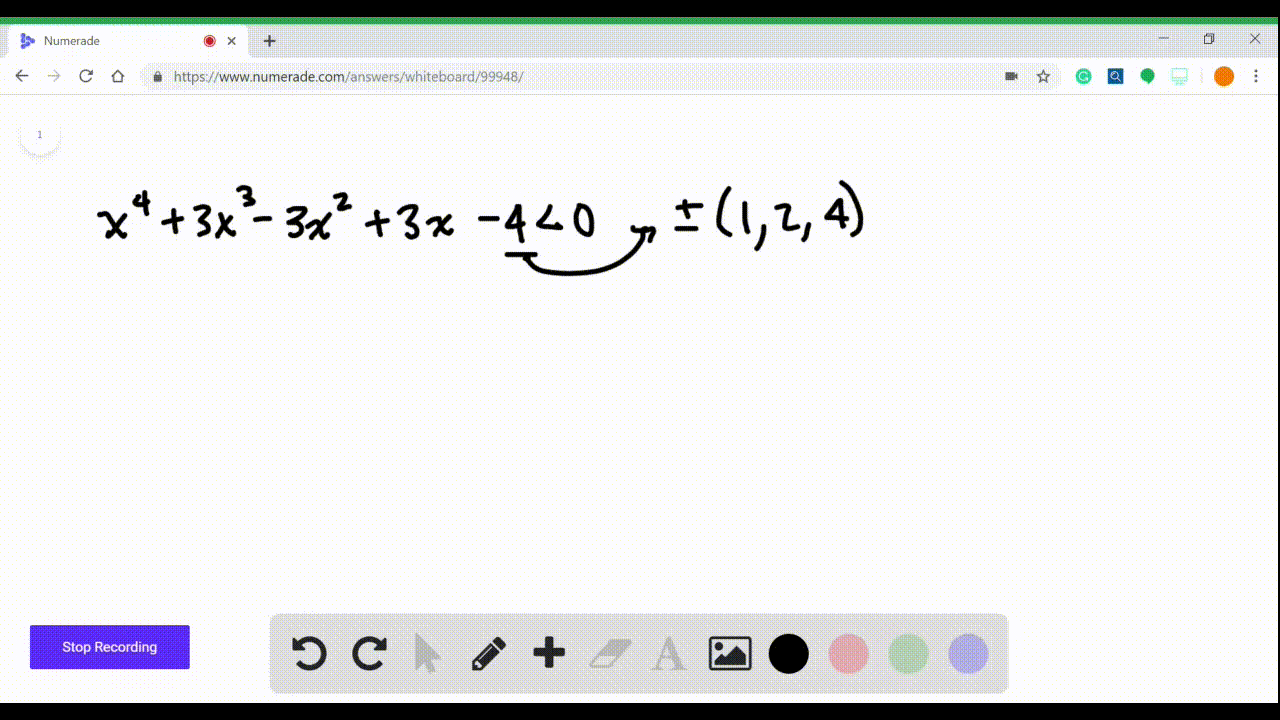Enroll in one of our FREE online STEM summer camps. Space is limited so join now!View Summer Courses### $3-16=$ Polynomial Inequalities Solve the inequal…

05:54Other Schools

Need more help? Fill out this quick form to get professional live tutoring.

Get live tutoring
Problem 13

$3-16=$ Polynomial Inequalities Solve the inequality.
$$x^{3}+x^{2}-17 x+15 \geq 0$$

## Discussion

You must be signed in to discuss.

## Video Transcript

So we're gonna win this inequality X cubed plus X squared minus 17 X plus 15 and were asked to find out when that's greater than or equal to zero. So the first thing we have to do is find the roots. So to do that, we have the factor. This And in order to do that, we have to use synthetic substitution and recognize that the possible factors are one plus or minus. Uh, plus or minus three. Let's remind us five and plus or minus 15. I'm gonna try one, and I can see that one works because one cubed plus one squared minus 17 times one plus 15 gives me zero. So I know that one is a route. So then I'm gonna divide by synthetic substitution. So I'm gonna go one and then 11 minus 17 and 15 and I'm gonna bring the one down first and then one times one is one. If I had to get to one. Times two is two minus 17 plus two is minus 15 times one is minus 15 and I get zero. So this thing factors now as X minus one, because one is the root on two X squared plus two x minus 15 is created that are equal to zero. And then that factors as X minus one times X close. Five X minus three is greater than or equal to zero. So that leaves me in terms of my number line with three possible routes, minus five one and then three. And all I do is I take a number that's less than that. So let's say four. Sorry not for, let's say, minus six. First, we're going there and I plug it into each of these. So minus six minus one is negative. Minus six plus five is negative and might have six. Minus three is negative. So I get three negatives, which makes this negative this interval. Negative. Then I plug in, Let's say, minus four in here, so minus four minus one is minus five. So it's negative. This is positive, though, cause it's minus four plus five and then minus four minus three is negative. So I get a positive here and then between one and three. I could put in two and I will get a negative and putting something bigger than three. Let's say four, I'll get a positive, and I'm confident that that is the case because these are all single roots. And if you have gone through the polynomial rational function chapter, the start of this chapter, you know that if it's a single room, it means it has to change. Sign when we look at the positive intervals are solution is going to be the interval from minus five upto one. It's positive and then from three to infinity. So that is our solution, so it's a bit of involved mathematics.